GCSE Maths Algebra

Maths Formulas

# Maths Formulas

Here we will learn about maths formulas, including what maths formulas are, how to use them and how to rearrange them. We will also look at special formulas involved in kinematics.

There are also maths formulas worksheets based on Edexcel, AQA and OCR exam questions, along with further guidance on where to go next if you’re still stuck.

## What are maths formulas?

Maths formulas are rules that are written connecting two or more variables. We can use maths formulas to work out the values of given variables, based on other known values.

To do this we substitute the values that we know into the formula and then calculate the value of the unknown.

For example, here is a rectangle with base b and height h.

The formula for the perimeter P of a rectangle is

P=2(b+h).

We can use this formula to find the value of the perimeter by substituting in the values for the base and height of the rectangle.

Other examples of maths formulas you may recognise include,

### What are maths formulas?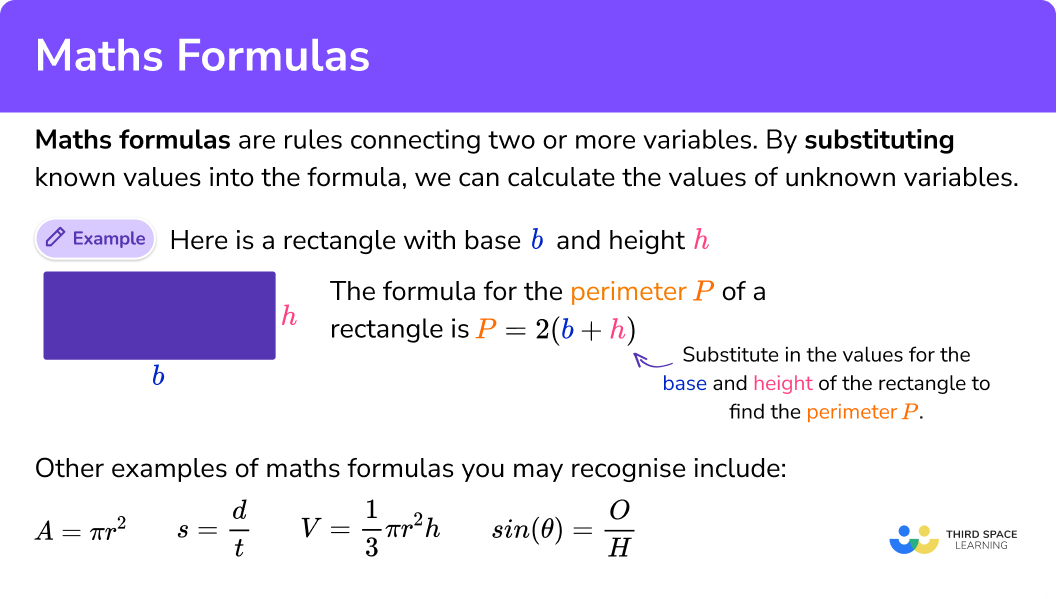### List of maths formula

These are the maths formulas you are most likely to encounter for GCSE.

Areas

Area of a parallelogram

A=bh \hspace{1.5cm} Where A is Area, b is base and h is height.

Area of a triangle

A=\frac{1}{2}bh \hspace{1.35cm} Where A is Area, b is base and h is height.

Area of a trapezium

A=\frac{1}{2}(a+b)h \hspace{.65cm} Where A is Area, a and b are the parallel sides and h is height.

Circles

Circumference of a circle

C=\pi d \hspace{1.6cm} Where C is circumference and d is diameter.

Area of a circle

A=\pi r^2 \hspace{1.55cm} Where A is Area and r is radius.

These formulas can be extended to give the following

\text{Arc length}=\frac{\theta}{360}\pi d \;\; and \;\; \text{Sector area}=\frac{\theta}{360}\pi r^2

Where \theta is the angle at the centre of the sector.

Volumes:

Volume of a cuboid:

V=lwh \hspace{1cm} Where V is volume, and l,w and h are the lengths of the 3 sides.

Volume of a prism:

V=\text{area of cross}-\text{section}\times \text{length}

Volume of a cylinder:

V=\pi r^2 h \hspace{1cm} Where V is volume, r is radius and h is height.

Angles in a polygon:

Sum of angles in a polygon:

S=180(n-2) \hspace{.5cm} Where S is sum and n is number of sides.

Compound measures:

Speed, distance, time

s=\frac{d}{t} \hspace{1.5cm} Where s is speed, d is distance and t is time.

Mass, density, volume

d=\frac{m}{v} \hspace{1.4cm} Where m is mass, d is density and v is volume.

Right-angled triangles:

Pythagoras’ theorem

a^2+b^2=c^2

Where c is the hypotenuse and a and b are the shorter sides of a right-angled triangle.

Trigonometry

\sin(\theta)=\frac{O}{H}. \hspace{1cm} \cos(\theta)=\frac{A}{H}. \hspace{1cm} \tan(\theta)=\frac{O}{A}.

Where O is the opposite side, A is the adjacent side and H is the hypotenuse.

Some formulas are for the Higher GCSE.

x=\frac{-b\pm \sqrt{b^2-4ac}}{2a}

Where a,b and c are coefficients of the quadratic equation to solve ax^2+bx+c=0.

Pyramids, Cones and Spheres

Volume of a pyramid

V=\frac{1}{3}\times \text{area of base} \times h \hspace{1cm} Where V is volume and h is height.

Volume of a cone

V=\frac{1}{3}\pi r^2 h \hspace{1cm} Where V is volume, r is radius and h is height.

Curved surface area of a cone

\text{CSA}=\pi rl \hspace{1cm} Where l is the slant length.

Volume of a sphere

V=\frac{4}{3}\pi r^3 \hspace{1.25cm} Where V is volume and r is radius.

Surface area of a cone

\text{SA}=4\pi r^2

Higher Trigonometry:

Area of a triangle

A=\frac{1}{2}ab \sin(C)

Sine rule

\frac{a}{\sin(A)}=\frac{b}{\sin(B)}=\frac{c}{\sin(C)}

Cosine rule

a^2=b^2+c^2-2bc \cos(A)

Where a,b and c are the sides of a triangle and A,B and C are the corresponding angles.

Coordinate geometry:

m=\frac{y_{2}-y_{1}}{x_{2}-x_{1}}

Where m is the gradient and (x_{1},y_{1}) and (x_{2},y_{2}) are coordinates.

Midpoint of two points is

(\frac{x_{1}+x_{2}}{2},\frac{y_{1}+y_{2}}{2})

The equation of a straight line is

y-y_{1}=m(x-x_{1})

### Using maths formulas

We can use maths formulas in a variety of ways.

For example, looking back to the rectangle above, we had the formula

P=2(b+h).

If we are given 2 of the values, we can use the formula to work out the third value.

Let’s look at a specific example.

Here b=13 and h=5 so using the formula for perimeter we can write,

\begin{aligned} P&=2(b+h)\\\\ P&=2(13+5)\\\\ P&=2\times 18\\\\ P&=36 \end{aligned}

We have calculated that the perimeter of the rectangle is 36cm.

### How to use maths formulas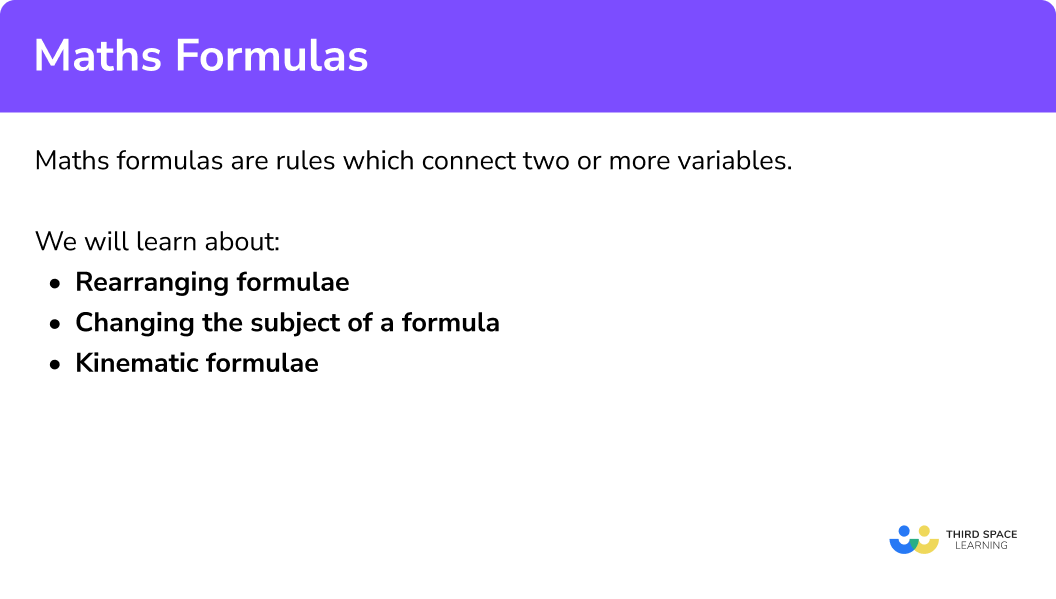### Rearranging formulas

Formulas can be rearranged in a similar way to equations.

To do this, we use inverse operations to make one chosen variable the subject of the formula. The subject of the formula is the single variable that is equal to everything else. i.e. the term by itself on one side of the equal sign.

For example, let’s rearrange P=2(b+h) to make h the subject.

Step-by-step guide: Rearranging formulae

### Kinematics formulas

Many of the formulas used at GCSE are about geometrical situations, for example to calculate area or perimeter. However, there are some formulas which are used to solve problems involving moving objects. Kinematics is the maths concerned with the movement of objects.

The 5 different kinematic formulas involve 5 different variables of motion.

The variables of motion are,

s= displacement

u= initial velocity

v= final velocity

a= acceleration

t= time

Velocity is speed in a given direction.

Displacement is the distance from the original position.

The five formulas are,

\begin{aligned} &v=u+at \\\\ &s=ut+\frac{1}{2}at^2 \\\\ &s=\frac{1}{2}(u+v)t \\\\ &v^2=u^2+2as \\\\ &s=vt-\frac{1}{2}at^2 \end{aligned}

The kinematic formulas are sometimes known as the suvat equations.

Step-by-step guide: Kinematic formulae

## How to use maths formulas

In order to work with maths formulas:

1. Use the formula given in the question.
2. Work carefully to answer the question, one step at a time.
3. Write the final answer clearly.

### Explain how to use maths formulas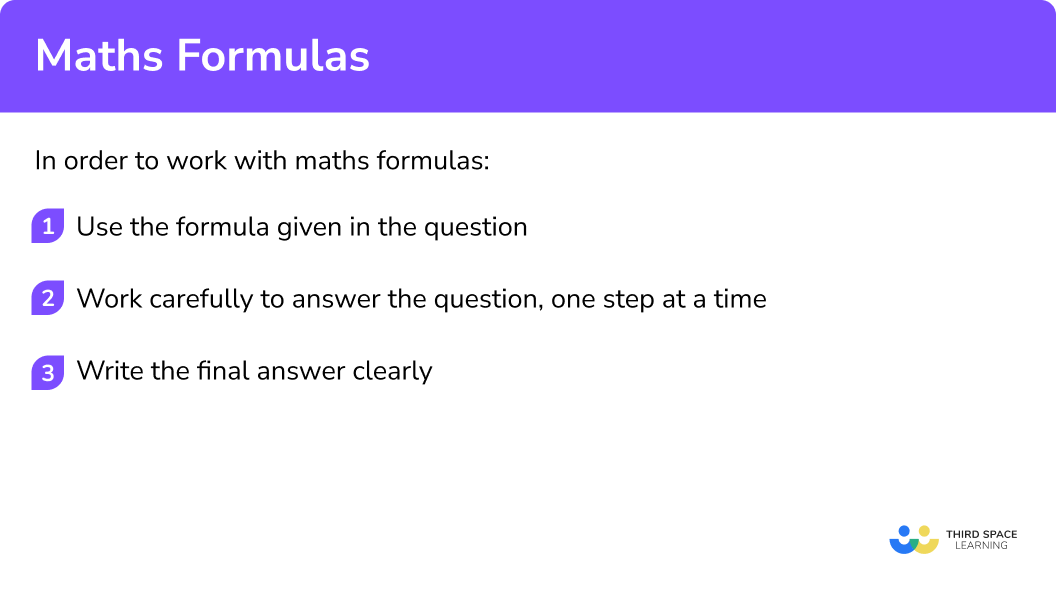## Maths formulas examples

### Example 1: using a maths formula

Given that F=\frac{9}{5}C+32 find F when C=50.

1. Use the formula given in the question.

The formula in the question is,

F=\frac{9}{5}C+32.

2Work carefully to answer the question, one step at a time.

Substitute the value that you know into the formula and work out the answer.

Here you know C=50.

\begin{aligned} F&=\frac{9}{5}C+32 \\\\ F&=\frac{9}{5}\times 50+32 \\\\ F&=90+32 \\\\ F&=122 \end{aligned}

### Example 2: using a maths formula

Given that D=\frac{M}{V}, calculate M when D=10 and V=40.

The formula in the question is

D=\frac{M}{V}.

This time you are given that D=10 and V=40. You can substitute these into the formula and then solve to find M.

\begin{aligned} D&=\frac{M}{V}\\\\ 10&=\frac{M}{40}\\\\ 10 \times 40 &=M\\\\ 400&=M \end{aligned}

### Example 3: rearranging equations

Make b the subject of the formula w=3ab+2 .

The formula in the question is

w=3ab+2 .

Rearrange the formula using inverse operations, one step at a time.

b=\frac{w-2}{3a} .

### Example 4: kinematics

A train starts from rest and accelerates at 2 \ m/s^{2}. Find its velocity after 20 seconds.

You may use v=u+at where

The formula given is

v=u+at.

You need to substitute the values into the formula and calculate the answer. The phrase starting at rest indicates that the initial velocity will be 0.

When answering kinematics questions, it can be helpful to start by writing down what you know.

Here you know that u=0, \ a=2 and t=20.

\begin{aligned} v&=u+at \\\\ v&=0+2\times 20 \\\\ v&=40 \end{aligned}

The final answer is 40 \ m/s. The units are consistent with the values in the original situation.

### Common misconceptions

• Take care with writing variables clearly

Neat writing is important. For example, the variables v and u can easily be muddled as they look similar and they both represent velocity in kinematic formulas.

• When we square root a number/variable the answer can be positive or negative

The square root of any value can be positive or negative. When taking a square root, use the plus/minus sign \pm to indicate this.

For example,

\begin{aligned} x^2&=ab-c \\\\ x&=\pm \sqrt{ab-c} \end{aligned}

### Practice maths formulas questions

1. Given that A= \frac{1}{2}bh, find A when b=24 and h=10.

A=68A=120A=240A=17Substitute the values into the formula and calculate the answer.

\begin{aligned} A&= \frac{1}{2}bh \\\\ A&= \frac{1}{2}\times 24\times 10\\\\ A&=120 \end{aligned}

2. Given the W=\frac{H}{A}+5, find the value of H when W=12 and A=4.

H=28H=8H=68H=43Substitute the values into the formula and calculate the answer.

\begin{aligned} W&=\frac{H}{A}+5\\\\ 12&=\frac{H}{4}+5\\\\ 7&=\frac{H}{4}\\\\ 28&=H \end{aligned}

3. Make t the subject of the formula,

h=3t-y.

t=\frac{h-3}{y}t=\frac{h+3}{y}t=\frac{h+y}{3}t=\frac{h-y}{3}Rearrange using inverse operations.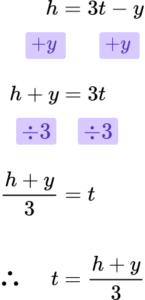4. Make x the subject of the formula,

a=\frac{x}{3}-b.

x=3a+bx=3a-bx=3(a-b)x=3(a+b)Rearrange using inverse operations.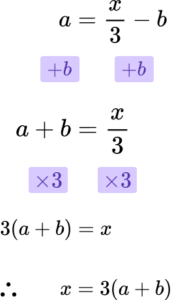5. Given that s=ut+\frac{1}{2}at^2, find s when u=10, \ a=3 and t=5.

s=57.5s=12.5s=87.5s=42.5Substitute the values into the formula and calculate the answer.

\begin{aligned} s&=ut+\frac{1}{2}at^2\\\\ s&= 10\times 5+\frac{1}{2}\times 3 \times 5^2\\\\ s&= 50+37.5\\\\ s&=87.5 \end{aligned}

6. A train is travelling at 28 \ m/s. It brakes for 4 seconds and it decelerates at 6 \ m/s^{2}. Calculate how far the train travels in those 4 seconds.

You may use the formula s=ut+\frac{1}{2}at^2.

Where s= displacement, \ u= initial velocity, \ v= final velocity, \ a= acceleration, \ t= time

64 \ m160 \ m124 \ m100 \ mSubstitute the given values into the formula. Note that a=-6 because the train is slowing down.

\begin{aligned} s&=ut+\frac{1}{2}at^2 \\\\ s&=28\times 4+\frac{1}{2}\times (-6) \times4^2 \\\\ s&=112-48 \\\\ s&=64 \end{aligned}

The answer will be 64 metres, as metres is consistent with the other units in the question.

### Maths formulas GCSE questions

(1 mark)

C is the formula.

(1)

( A is an equation, B is an identity, D is an expression.)

2. Rearrange x=my-8 to make y the subject.

(2 marks)

x+8=my

(1)

y=\frac{x+8}{m}

(1)

3. For the following question you may use the formula v=u+at where

A motorbike is travelling along a road at a velocity of 11 \ m/s. The motorbike accelerates at 3 \ m/s^2 for 4 seconds. Find the final velocity.

(2 marks)

v=11+3\times 4

(1)

v=23 \ m/s

(1)

## Learning checklist

You have now learned how to:

• Use mathematical formula
• Rearrange equations and formula
• Use kinematic formulas

## Still stuck?

Prepare your KS4 students for maths GCSEs success with Third Space Learning. Weekly online one to one GCSE maths revision lessons delivered by expert maths tutors.

Find out more about our GCSE maths tuition programme.